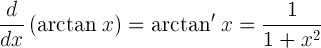# Derivative of arctan

What is the derivative of the arctangent function of x?

The derivative of the arctangent function of x is equal to 1 divided by (1+x2)